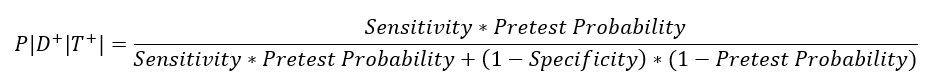# Principles of Diagnostic Testing Series | Part 4

## Disease Probability

Probability is the chance that some event will happen. With disease, probability can be thought of as out a group of individuals exposed to a disease, how many will become infected. Diagnostic tests do not always appropriately classify an individual as diseased or not diseased and test predictive values are used to assess the probability that the test result is true. Predictive value depends upon diagnostic test sensitivity and specificity and the pre-test probability of disease. Sensitivity and specificity are characteristics of the diagnostic test. Diagnostic sensitivity is the proportion of individuals with the disease that test positive. Diagnostic specificity is the proportion of individuals without the disease that test negative. Disease pre-test probability is the clinical suspicion of a disease in an individual. Individuals with clinical signs consistent with a particular disease will have a higher pre-test probability than those without clinical signs.

Positive predictive value (PPV) is the probability that a diagnostic test positive individual has the disease. High positive predictive values indicate that individuals that test positive on a diagnostic test have a high probability of having the disease and can be used to rule in a condition.Negative predictive value (NPV) is the probability that a diagnostic test negative individual does not have the disease. High negative predictive values indicate that individuals that test negative on a diagnostic test have a high probability of not having the disease and can be used to rule out a condition.